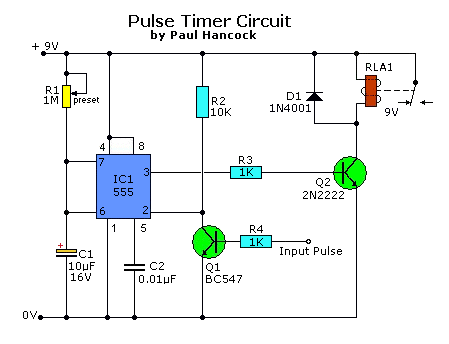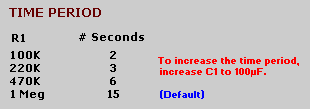## 555 Pulsni Timer Oscillator

555 Pulsni Timer OscillatorLista dijelova:
R1 = 1 Meg, Preset Pot
R2 = 10K
R3,R4 = 1K

C1 = 10uF, 16V, electrolytic
C2 = 0.01uF, ceramic

Q1 = BC547 (Gen Purp NPN) or 2N3904
Q2 = 2N2222 (Hi Current NPN, up to 800mA)
D1 = 1N4001 (Gen Purp Si)
IC1 = 555 (Lo-Power version)
RLA1 = Relay, 9V (amps of your choice)Izvor: tonys website